The Matrix，黑客帝国。

### 为什么说矩阵是机器学习的核心？

from sklearn.datasets import load_iris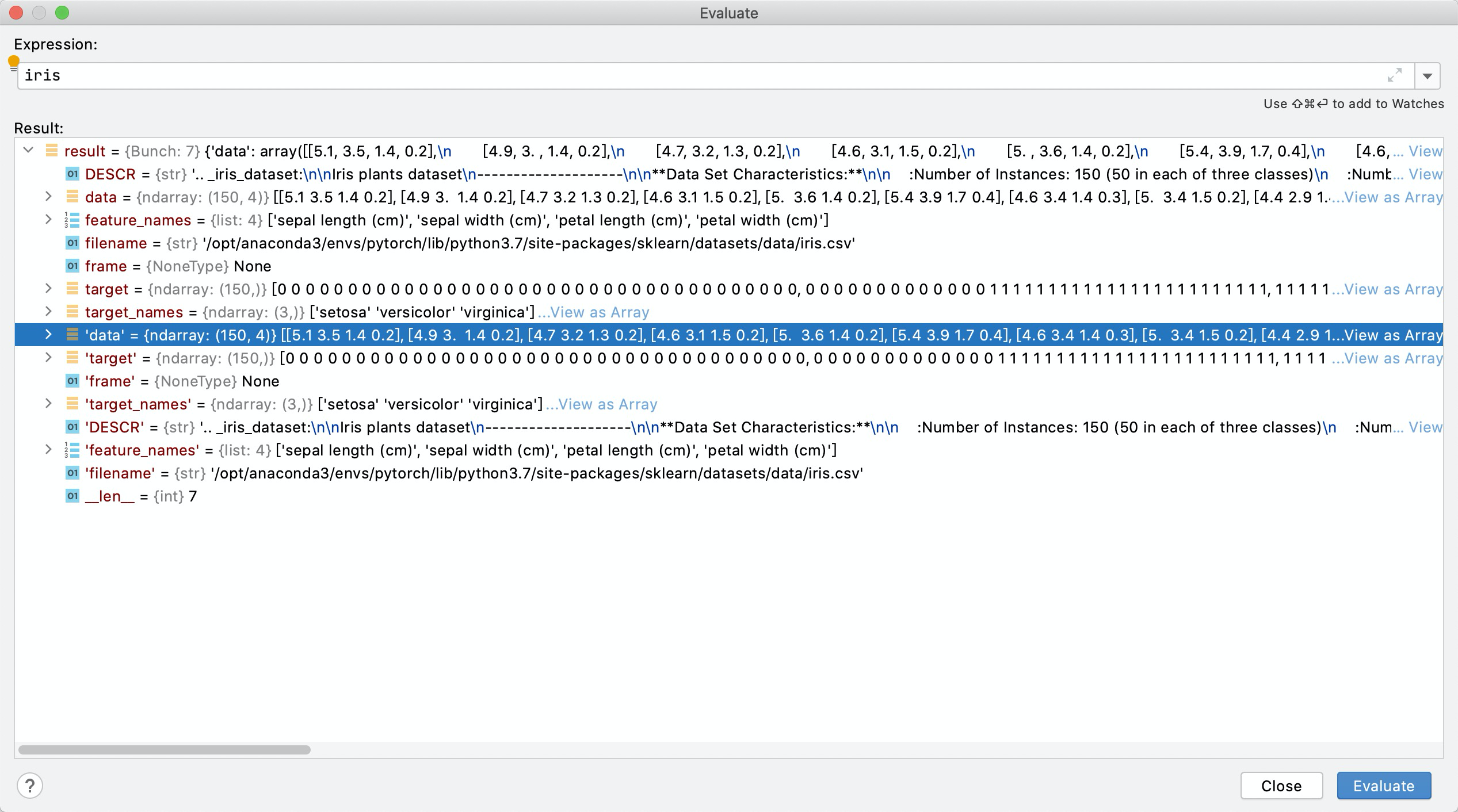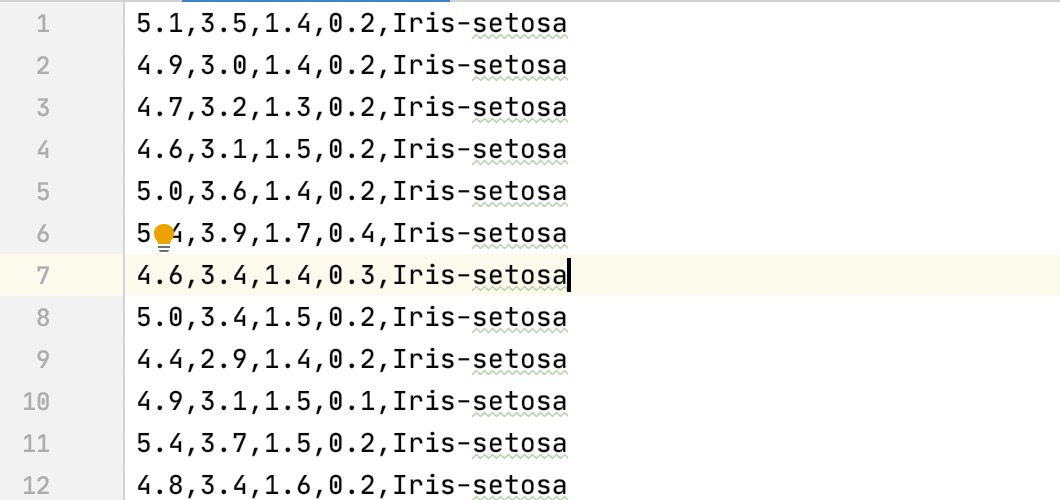from matplotlib import pyplot

setosa_sepal_len = iris.data[:50, 0]
setosa_sepal_width = iris.data[:50, 1]

versi_sepal_len = iris.data[50:100, 0]
versi_sepal_width = iris.data[50:100, 1]

vergi_sepal_len = iris.data[100:, 0]
vergi_sepal_width = iris.data[100:, 1]

pyplot.scatter(setosa_sepal_len, setosa_sepal_width, marker = 'o', c = 'b',  s = 30, label = 'Setosa')
pyplot.scatter(versi_sepal_len, versi_sepal_width, marker = 'o', c = 'r',  s = 50, label = 'Versicolour')
pyplot.scatter(vergi_sepal_len, vergi_sepal_width, marker = 'o', c = 'y',  s = 35, label = 'Virginica')
pyplot.xlabel("sepal length")
pyplot.ylabel("sepal width")
pyplot.title("sepal length and width scatter")
pyplot.legend(loc = "upper right")
pyplot.show()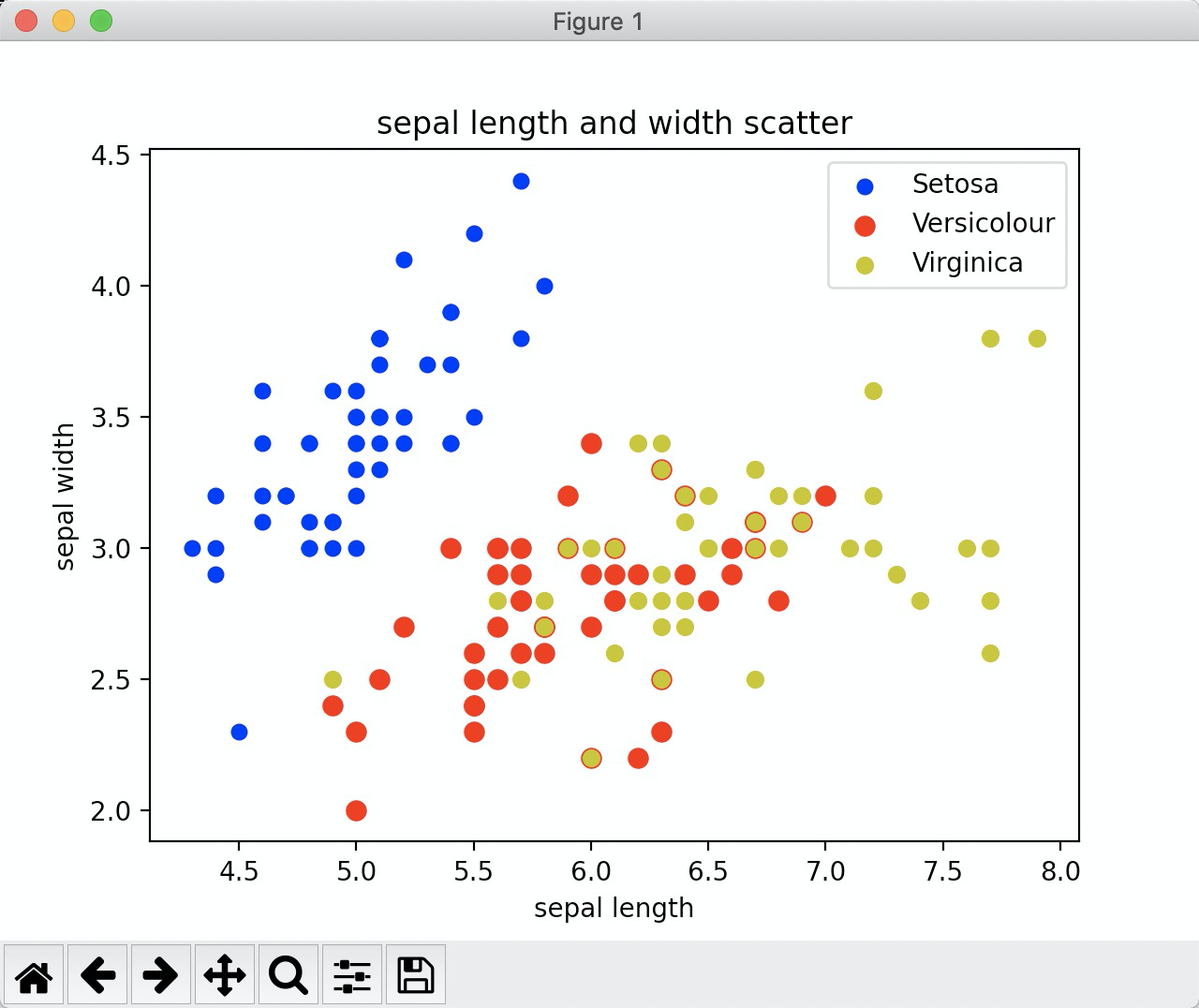### 机器学习分类

##### 1. 监督学习

http://yann.lecun.com/exdb/mnist/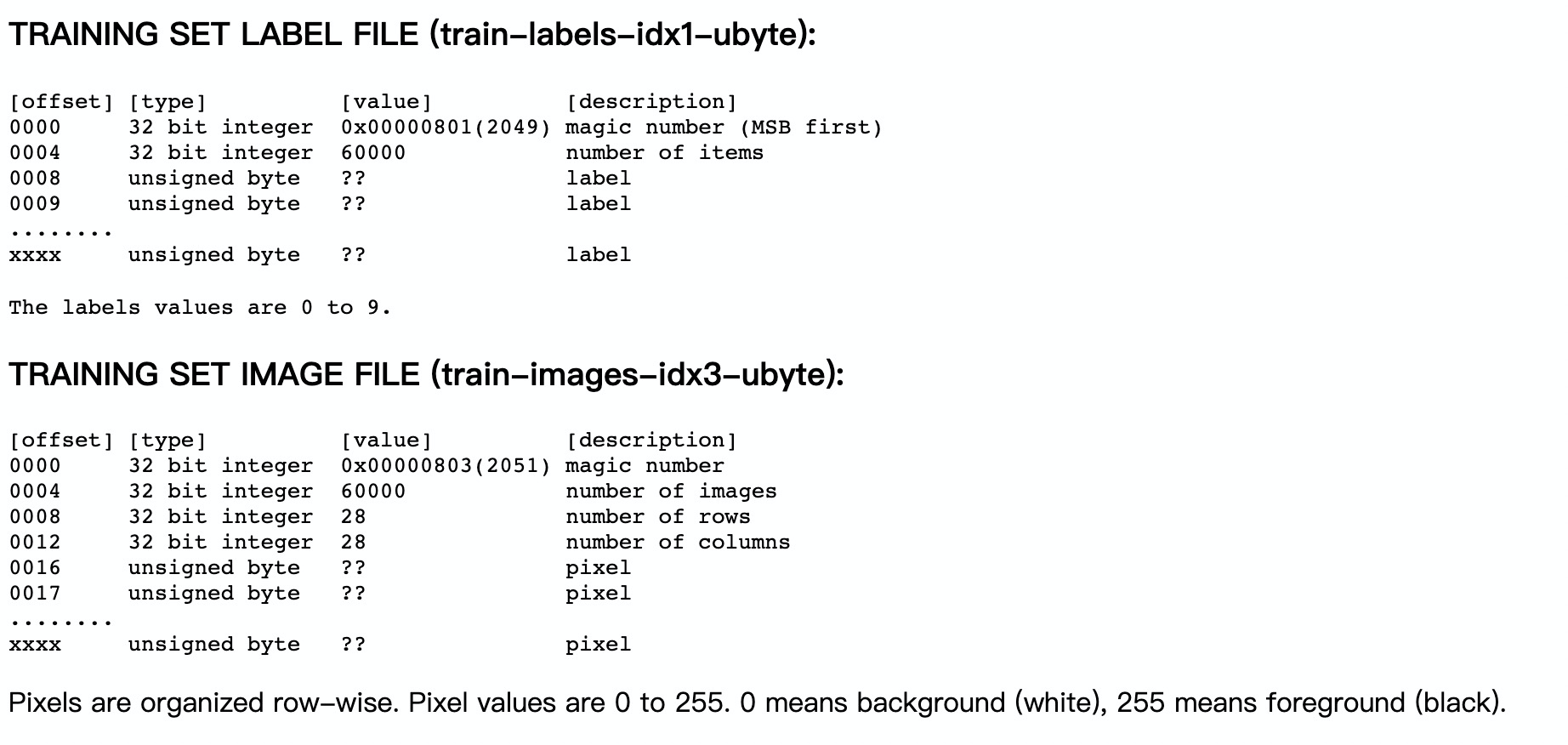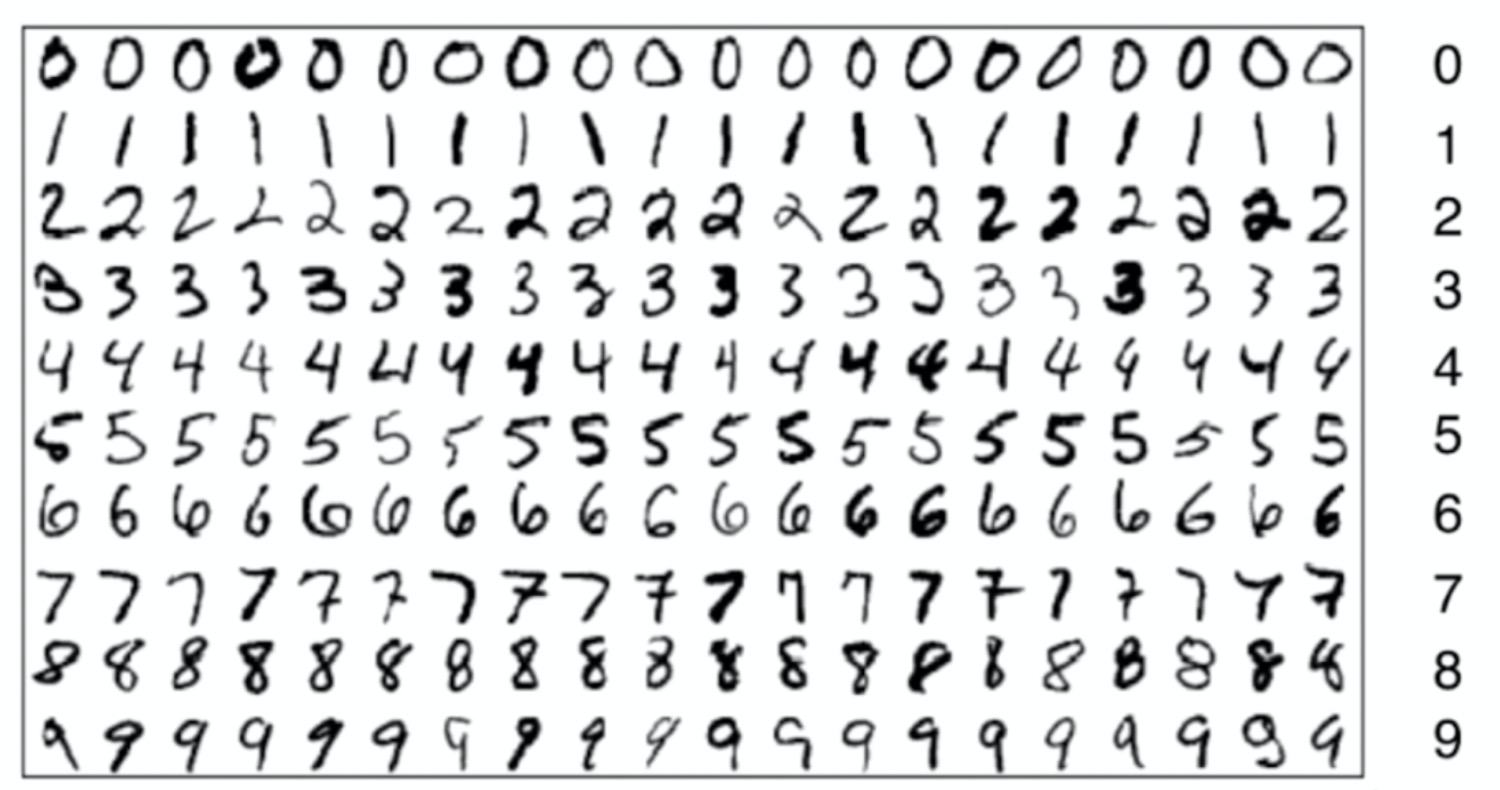##### 4. 增强学习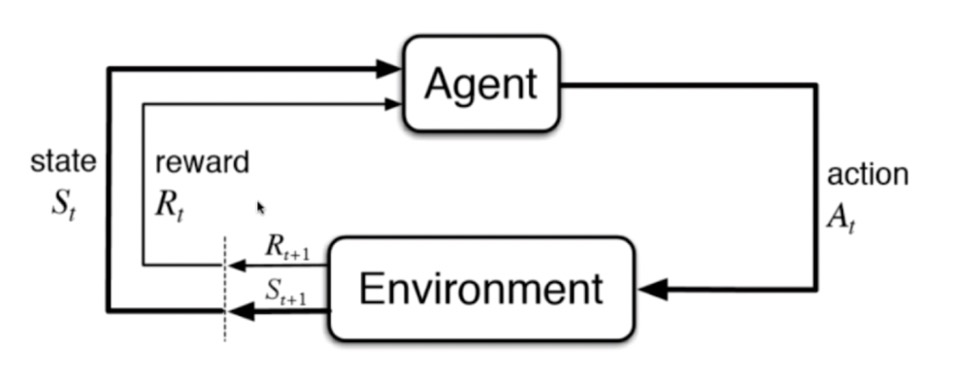## 待完成

K邻近监督学习

••